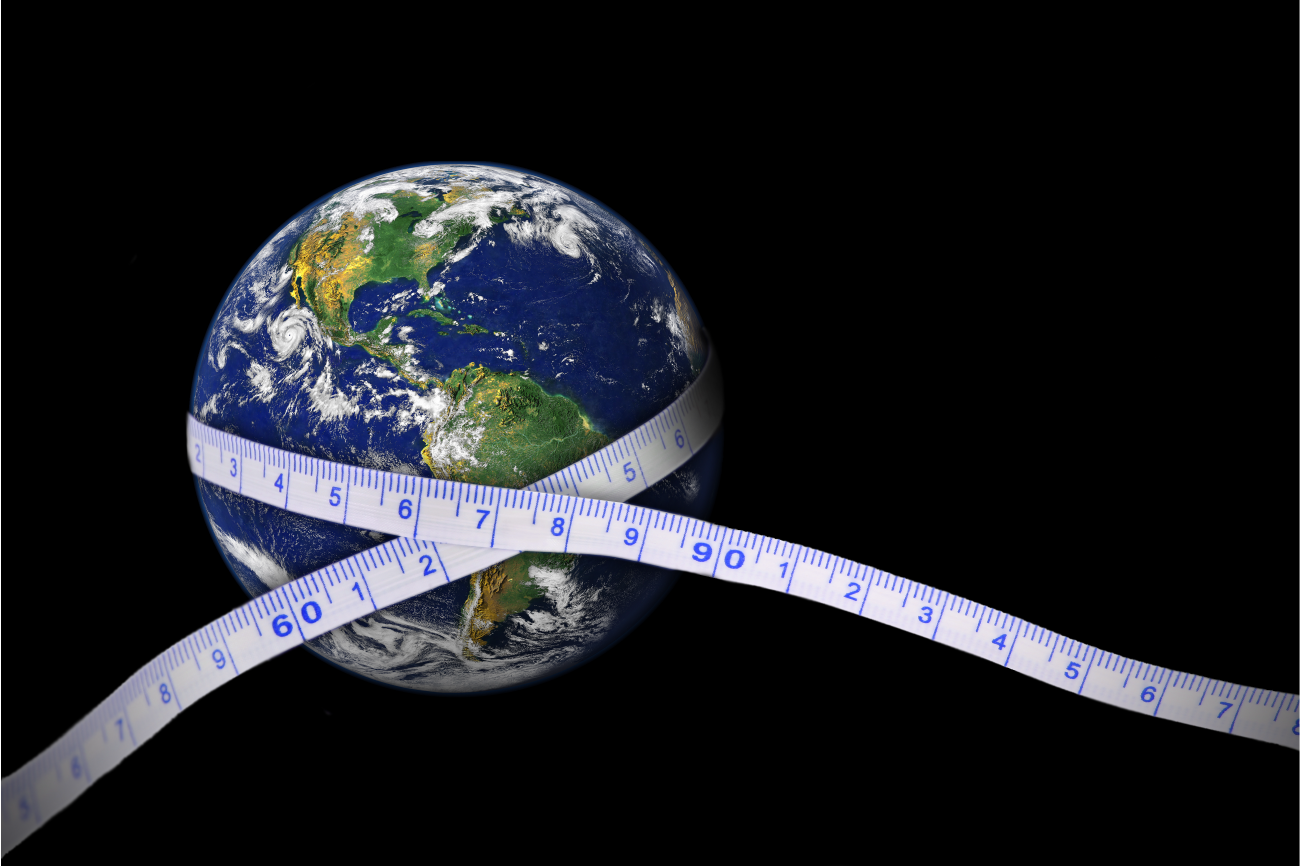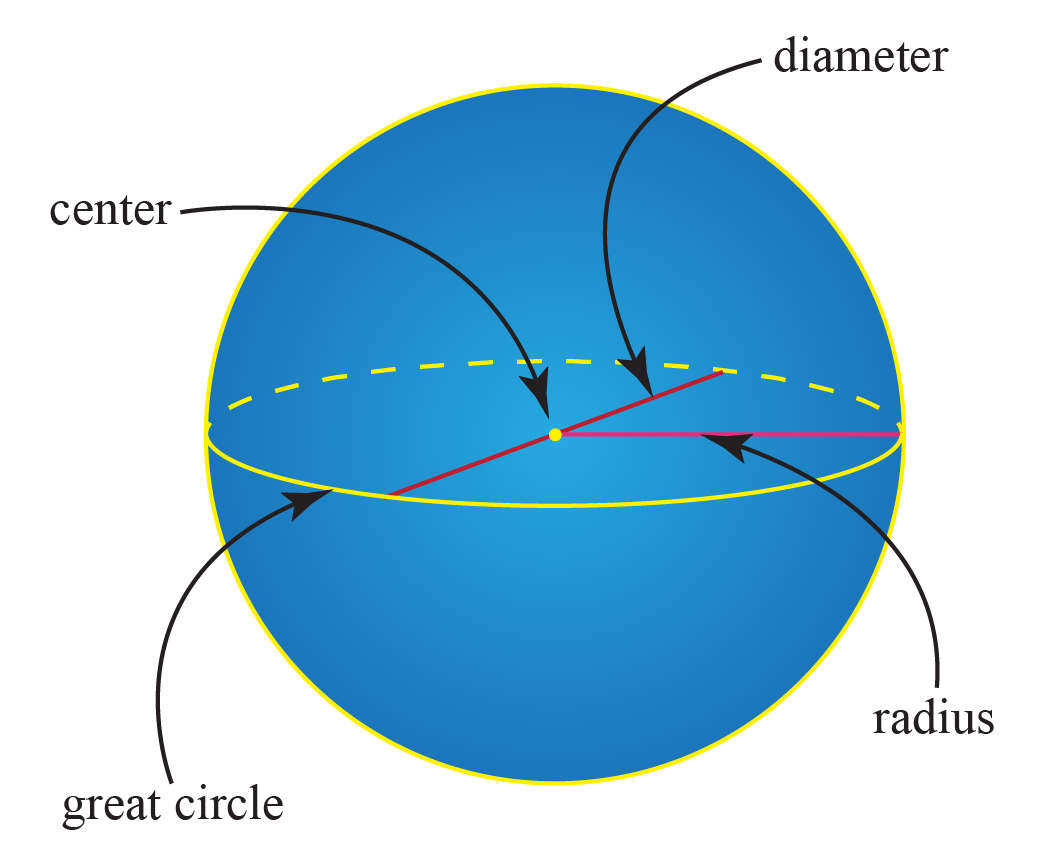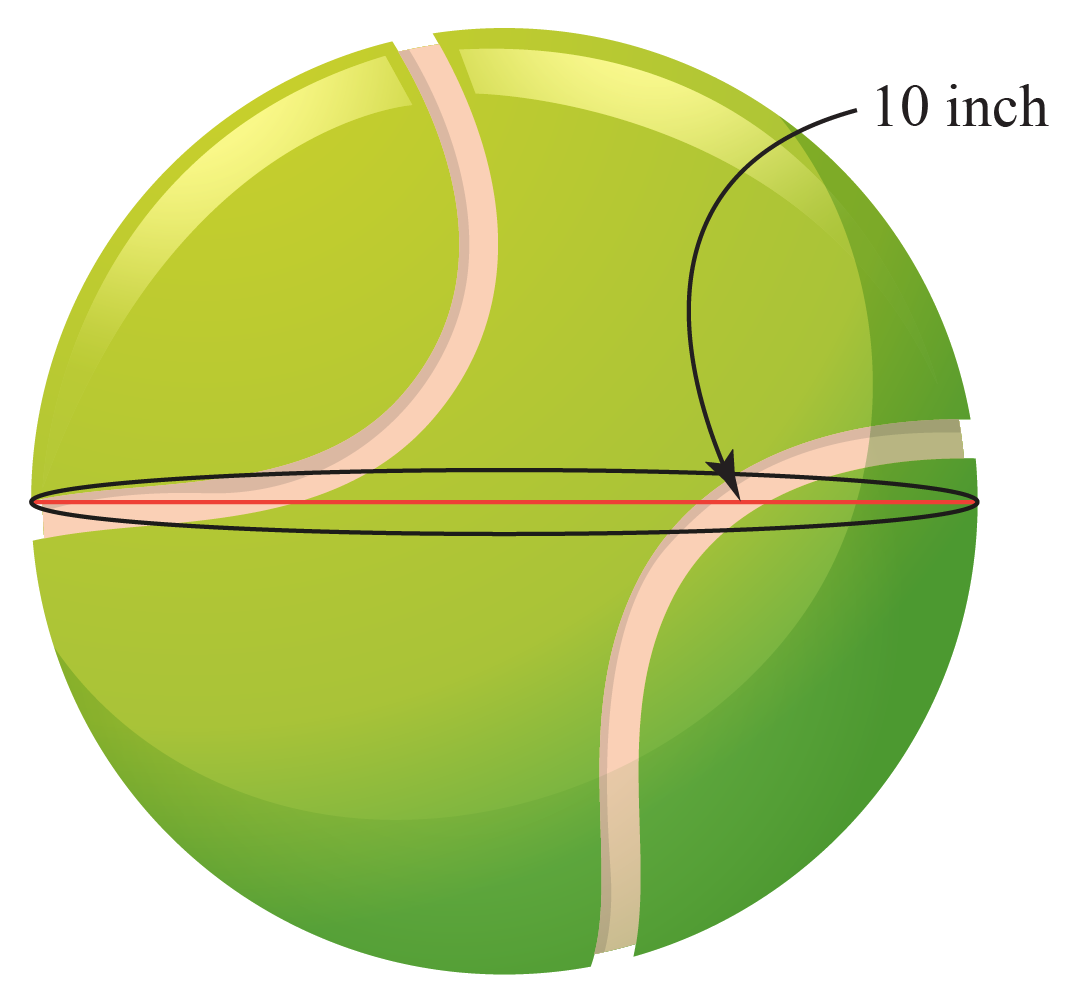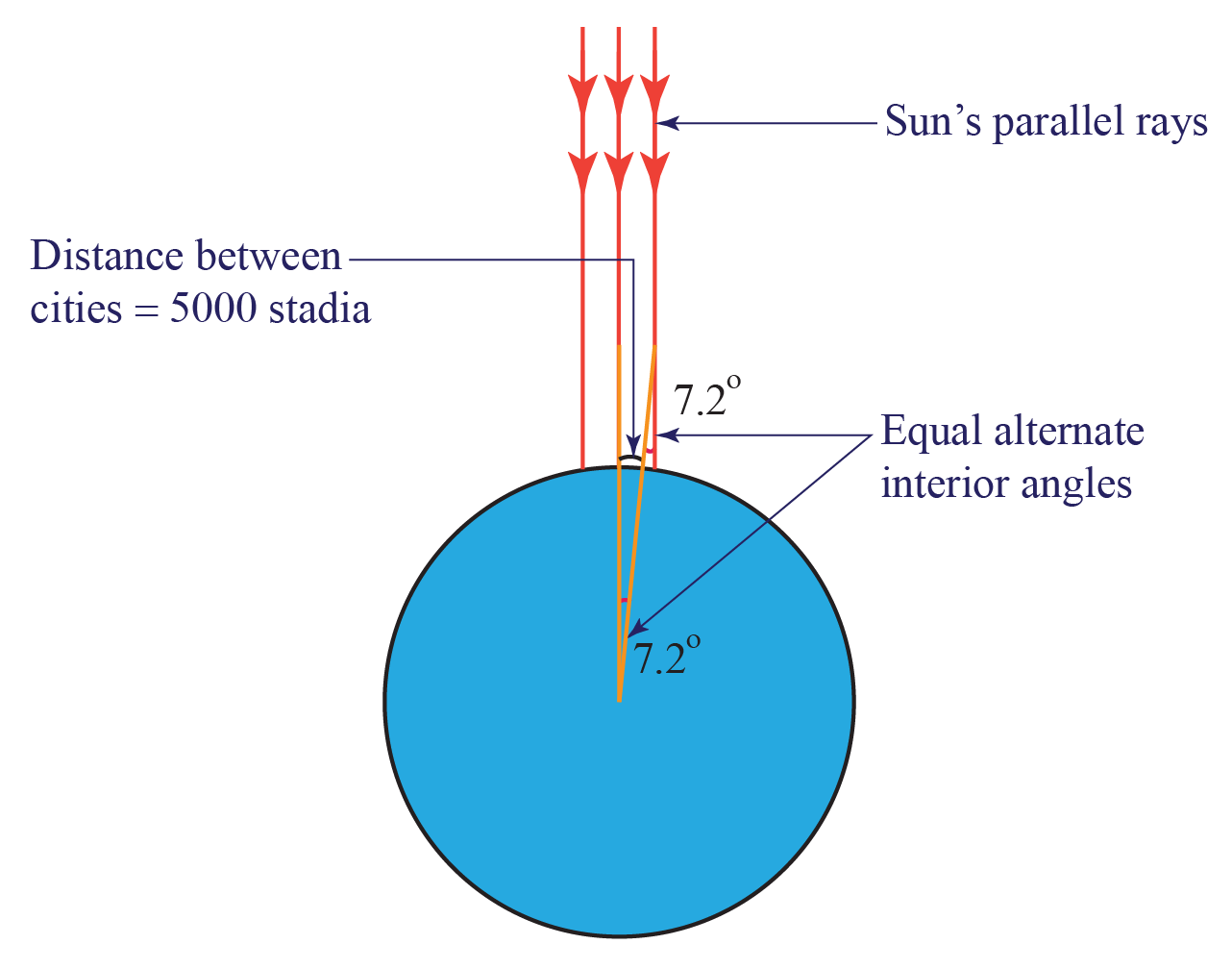# Circumference of the Earth

Circumference of the Earth
Go back to  'Circles'

Joey and Rachel were standing at the Baltimore Harbour trying to find a day-to-day example that proved that the Earth was round. They had read it in textbooks, but they wondered if there was any living example.

Just then, their friend Ross came along. Both Joey and Rachel explained the situation to Ross, who asked them to look at the boats at a distance.

The boats, Ross explained, would disappear from the horizon, which meant that the Earth was a gigantic sphere.

Ross then showed Joey and Rachel a basketball he was carrying. There was an ant on it.The basketball is a relatively small object for us, Ross explained. But for the ant, it's a flat path.

"The ant won't realize that it is going around a circle. If you compare the size of the ant to the basketball, versus us to the size of Earth, then we are much smaller," Ross explained.

Planet Earth is spherical in shape, and therefore will have a radius, a diameter, a total surface area, as well as a circumference.

In this chapter, we will learn about the circumference of Earth. Once we get the basics right, we will learn about the circumference of the earth in miles, the circumference of the earth in km, the circumference of the earth in meters, the diameter of the earth in the concept of the circumference of the earth.

Check out the interactive simulations to know more about the lesson and try your hand at solving a few interesting practice questions at the end of the page.

## What is the Circumference of the Earth?

Earth's circumference is the length around the earth.

If you are standing at a point on the earth and move around the earth in a straight line, and reach the same point again, then the distance covered by you is the circumference of the earth.

However, the Earth’s circumference is different depending on how you measure it.

The Earth circumference around the equator is 40,075 km, which is around 24,887 miles. And if you measure it from the north pole to the south pole, it is 40,007 km, which is approximately 24,806 miles.## What is the General Formula For the Circumference of a Sphere?

A sphere doesn't have a circumference but the circular cross-section of a sphere does have a circumference.

But knowing the location of the cross-section is important as the length of the radius changes as we move away from the center of the sphere.

For that, we need to find out the great circle of the sphere, it can be located and well understood from the image shown below.Now, the circumference of the great circle or the circular cross-section is the distance around it and can be referred to as the circumference of the sphere.

Mathematically, it can be calculated by the formula:

 $$\text {Circumference} = 2 \, \pi \, \text { R or } \pi \, \text D$$

Where R is the radius of the sphere and D is the diameter of the sphere(if the circular cross-section is taken at the exact center of the sphere).

### Example

Consider a tennis ball whose great circle has a diameter of 10 inches.Then the circumference(C) of the tennis ball will be:

$C = \pi \, D = \pi \, 10 31.4 in$

 $$\therefore C = 31.4in$$

## How to Calculate the Circumference of the Earth?

Earth's circumference can be found out by various methods which are explained below:

Method 1: Earth's circumference can be found out by using the geometric formula that was used over 2,000 years ago.

Explanation

Eratosthenes, the head librarian in Alexandria, Egypt, in 200 B.C. estimated the earth's circumference using the following method:

He knew the following things and based his calculations on them accordingly:

1. On the longest day of the year, the sun would be directly above Syene, Egypt, or the angle of the sun rays would be 0°.

2. The distance of the city of Alexandria from Syene, Egypt, 5000 stadia.

He calculated the angle of rays in the city of Alexandria, which was measured as 7.2°.He then used the following relation:

"Ratio of the angle of the arc to the complete angle = Ratio of the length of the arc to the circumference"

$\implies \dfrac {7.2°}{360°} = \dfrac {5000}{C}$

He arrived at the estimated value of 25,000 stadia which almost equals 28,725 miles.

This estimated value is very close to the actual value of 24,901miles which is quite an astonishing achievement.

Method 2: We can also find out the circumference of the earth by using the direct formula for the circumference.

Explanation

$\text {Circumference} = 2 \, \pi \, \text {R or } \pi \, \text D$

Where R is the radius of the sphere and D is the diameter of the sphere.

But because of the recent technological advancement, we now know that Earth is not a perfect square but an oblate spheroid, i.e., has an equatorial diameter greater than the polar diameter(the equatorial diameter of the earth is 7,926 miles and the polar diameter of the earth is 7,900 miles).

Hence circumference of the earth will be different for both the cases mentioned above.

Therefore, the Circumference of the earth around the equator is:

$\text C_E = \pi \times 7926 = 24,887.64 \text { miles}$

And the Circumference of the earth around the poles is:

$\text C_P = \pi \times 7900 = 24,806 \text { miles}$Important Notes
• The location of the cross-section taken on the sphere is important as the length of the radius changes as we move away from the center of the sphere
• The great circle of a sphere is the circle of the largest diameter that can be drawn in the sphere.
• Things Eratosthenes assumed that Syene was precisely on the Tropic of Cancer and Syene and Alexandria are on the same meridian.

## Solved Examples

 Example 1

Charlie was given a problem to calculate the circumference of the planet Mercury. Can you help him with this?

It is given that the diameter of the planet Mercury is 3,031 miles.

Solution

We know,

The diameter of the planet Mercury = 3,031 miles

And $\text {Circumference (C)} = \pi \, \text D$

On putting the value of D in the circumference formula, we get $\text C = \pi \times 3031$

 $$\therefore$$ Circumference of Mercury  = $$9517.34 \text{ miles}$$
 Example 2

Trevor wants to know the ratio of the circumference of the sun to the circumference of the earth. Do you know how to solve this problem?

It is given that the diameter of the sun is 865,370.0 miles and the diameter of the earth  7,917.5 miles.

Solution

Diameter of the sun = 865,370.0 mi

Diameter of the earth = 7,917.5 mi

We know,$\text {Circumference (C)} = \pi \, \text D$

Circumference of the sun  = $$\pi \times 865,370.0 = 27,17,261 mi$$

Circumference of the earth = $$\pi \times 7,917.5 = 24,860.95 mi$$

Ratio of the circumference  = $$\dfrac {2717261}{24860.95}$$

 $$\therefore \text { Ratio of the circumference } = 109.29$$
 Example 3

The ratio of the angle subtended by the arc to the complete angle was known to Fiona. She wants to know the circumference of the great circle of the sphere whose arc she has given.

The length of the arc is given as 490 yards, and the ratio of the angles is 1/7°.

Solution

We know,

The ratio of the angle subtended by the arc to the complete angle = ratio of arc length to the circumference of the complete circle.

$\dfrac17 = \dfrac {490}{ \text C }$

 $$\therefore C = 3430 \text{ yards}$$
 Example 4

The circumference of the earth is 24,860.95 mi. What is the circumference of the earth in feet? (use 1 mile = 5280 feet)

Solution

Given,

Circumference of the earth = 24,860.95 mi

Since,

$1 \text { mi } = 5280 \text { ft}$

 $$\therefore$$ circumference of the earth  = $$13,12,65,605 \text { feet}$$
 Example 5

If for an oblate spheroid, the ratio of equatorial diameter to the polar diameter is 8. What will be the ratio of the circumference of the great circles of the sphere around the equator and the poles?

Solution

The ratio of the diameters = 8

Since,

The ratio of the circumference = The ratio of the diameters

Hence,

 $$\text { The ratio of the circumference }= 8$$Challenging Questions
• Do you know why the earth's equatorial diameter is greater than the polar diameter?

## Interactive Questions

Here are a few activities for you to practice.

Select/type your answer and click the "Check Answer" button to see the result.

## Let's Summarize

The mini-lesson targeted the fascinating concept of the Earth's circumference. The math journey around the circumference of the Earth starts with what a student already knows, and goes on to creatively crafting a fresh concept in the young minds. Done in a way that not only it is relatable and easy to grasp, but also will stay with them forever. Here lies the magic with Cuemath.

At Cuemath, our team of math experts is dedicated to making learning fun for our favorite readers, the students!

Through an interactive and engaging learning-teaching-learning approach, the teachers explore all angles of a topic.

Be it worksheets, online classes, doubt sessions, or any other form of relation, it’s the logical thinking and smart learning approach that we, at Cuemath, believe in.

## 1. Who was the first to calculate the circumference of the earth?

In 200 B.C, Eratosthenes calculated the circumference of the earth b using simple geometry.

He evaluated the ratio of the angle subtended by the arc between Alexandria, Egypt, and Syene, Egypt, to the complete angle with the ratio of the length of the arc to the circumference of the earth.

## 2. Where is the Earth's circumference greatest?

As the shape of the earth is an oblate spheroid, the diameter is different at the equator and at the poles.

Since the diameter at the equator is greater than the diameter at the poles, hence the circumference of the great circle at the equator is the greatest.

## 3. What is so interesting about the Earth’s circumference?

Circumference of the earth is not the same everywhere.

As the shape of the earth is an oblate spheroid, the diameter is different at the equator and at the poles.

Hence, the circumference of the earth is different at the equator and the poles.

More Important Topics
Numbers
Algebra
Geometry
Measurement
Money
Data
Trigonometry
Calculus